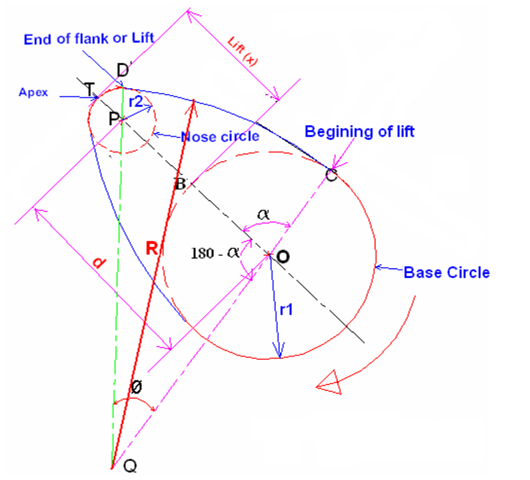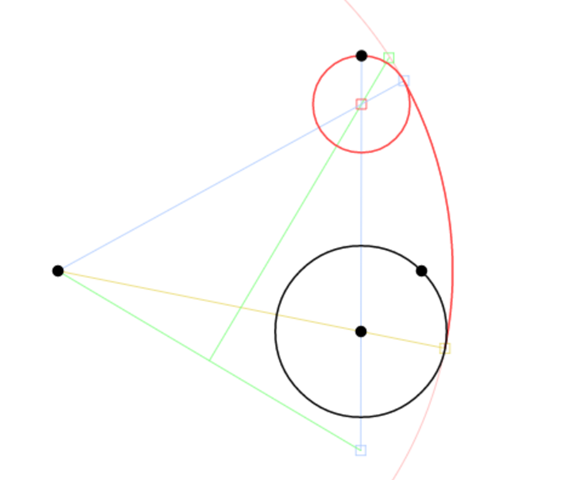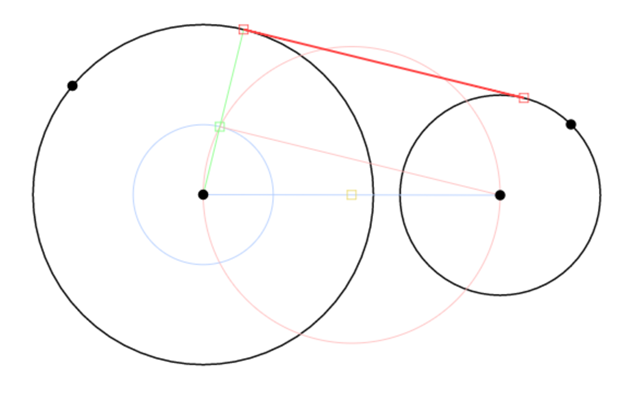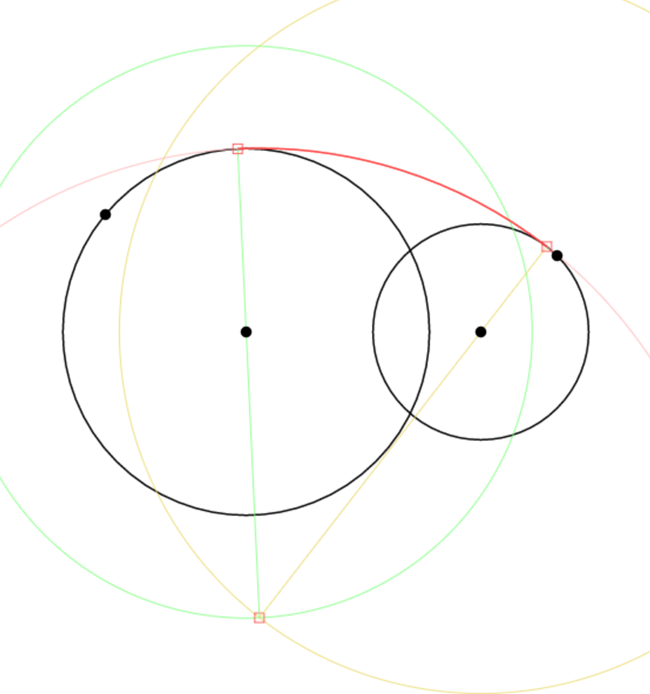I am writing software to simulate the operation of a cam and follower. I’ll add this to the Linkage program as soon as the details are worked out and the code is known to work right.

The first type of cam I want to use is a typical engine valve cam. I am not really sure if this is typical but it seems to be.##### Someone’s Cam Shape Example

I “borrowed” the picture above to show how this type of cam looks. The flank radius could be infinity making the flank flat, and I would handle that situation in my simulator quite easily.

The first thing I needed to do for a curved tangent was to understand how I can construct the cam image. With the cam image, the simulator would just do some circle-circle or circle-line intersectioning to simulate the follower movement.##### My First Cam

The first cam I constructed accepts a center point of the flank radius and a center point and radius for the base circle. The end of the nose circle is mutable. the center of the nose is computed. This is sort of how people design cam lobes in the real world. The cam is constructed from these various values and the picture above is what I get. See it here.

The problem with that cam above is that the center of nose will be moved around as the simulator rotates the cam. The cam construction above is no good because the nose center is computed. That black spot out at the end of the cam is used to change the angle of the cam but the nose radius changes with it instead of just the angle of the entire construction.##### Simple Circles Tangent

Before I worked on making a curved tangent to two circles, I first needed to do the construction of a straight tangent. It is an easy process where a few circles are used to find a line parallel to the final tangent line and it is then moved into place. The image shows the construction of the various parts, resulting in the dark red line being computed.

That was easy. But how to make that a curve? I had no idea and for some strange reason, I was unable to find a website that described how to do it. I found sites to find similar stuff but nothing that took a tangent curve radius and found the center of it based on two starting circles. The first thing I did was draw a picture of what I wanted using a compass and straight edge. I drew in many tangent curves at various radii by guessing at their locations and then plotted (hand drew) a curve through those center points. There was no way I could figure out the equation that described that curve because I am, frankly, not a math person. But without math, I suddenly realized what to do, because those center points all had one thing in common; they are all a known distance from the center of each of the starting circles.

Ah ha! With a known distance, I can simply construct two circles, one for each of the starting circles, and the intersection of those new circles is the center of the tangent curve.

Ugh, too much text. Here’s a picture:##### Curved Tangent Construction

The green circle is drawn around one of the start circles and it’s radius is the tangent radius minus the start circle radius. The yellow circle is constructed in the same way around the other start circle. The red square where they intersect is the center of the tangent radius. It’s as simple as that. See it working here.

The green and yellow circles can be smaller than the starting circles that they are created for. This works in all cases where one circle is not fully enclosed in the other. This will work for the cam simulator because the centers of the start circles and the start circle radii, as well as the tangent radius, will be the only known values. The yellow and green lines are used to find the start and end of the arc that is the curved tangent. Or should I say “tangent arc”?